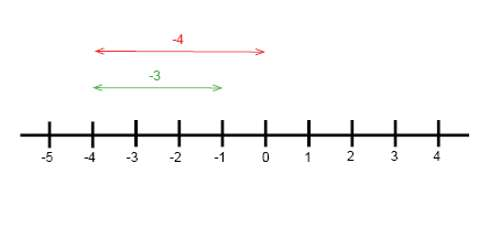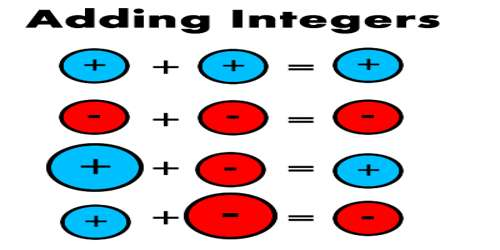Mathematic

# Addition Equations with four Digit IntegersAddition equations with four digit integers

An equation is a mathematical statement that has an expression on the left side of the equals sign (=) with the same value as the expression on the right side. An example of an equation is 2 + (- 6) = – 4.

An equation is a mathematical statement such that the expression on the left side of the equals signs (=) has the same value as the expression on the right side. An example of an equation is 2000 + (- 6000) = – 4000.

One of the terms in an equation may not be know and needs to be determined. Often this unknown term is represented by a letter such as x. (e.g. 2000 + x = – 4000).

The 4 digit number is 4569.

• The 9 is representing the ones place of number.
• The 6 is representing the tens place of number.
• The 5 is representing the hundreds place of number.
• The 4 is representing the thousands place of number.

Adding the 4 digit number combination is used the math symbol is +. Add the 4 digit number is used two operands and one operator.

The example is 7894 + 5692 = 13586.

7894 and 5692 is 4 digit operands.

The + is symbol of addition.

Subtract the 4 digit number combination is used the math symbol is -. Subtract the 4 digit number is used the one minuend, one subtrahend. The example is 7412 – 6392 = 1020

The solution of an equation is finding the value of the unknown x. To find the value of x we can use the subtractive equation property which says: The two sides of an equation remain equal if the same number is subtracted from each side. We may also use the additive equation property which says: The two sides of an equation remain equal if the same number is added to each side.Example:

– 5000 + x = 4000

– 5000 + x + 5000 = 4000 + 5000

0 + x = 9000

x = 9000

Check the answer by substituting the answer (9000) back into the equation.

– 5000 + 9000 = 4000

Information Source: